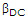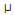# Electronics - Bipolar Junction Transistors (BJT)

## Why Electronics Bipolar Junction Transistors (BJT)?

In this section you can learn and practice Electronics Questions based on "Bipolar Junction Transistors (BJT)" and improve your skills in order to face the interview, competitive examination and various entrance test (CAT, GATE, GRE, MAT, Bank Exam, Railway Exam etc.) with full confidence.

## Where can I get Electronics Bipolar Junction Transistors (BJT) questions and answers with explanation?

IndiaBIX provides you lots of fully solved Electronics (Bipolar Junction Transistors (BJT)) questions and answers with Explanation. Solved examples with detailed answer description, explanation are given and it would be easy to understand. All students, freshers can download Electronics Bipolar Junction Transistors (BJT) quiz questions with answers as PDF files and eBooks.

## Where can I get Electronics Bipolar Junction Transistors (BJT) Interview Questions and Answers (objective type, multiple choice)?

Here you can find objective type Electronics Bipolar Junction Transistors (BJT) questions and answers for interview and entrance examination. Multiple choice and true or false type questions are also provided.

## How to solve Electronics Bipolar Junction Transistors (BJT) problems?

You can easily solve all kind of Electronics questions based on Bipolar Junction Transistors (BJT) by practicing the objective type exercises given below, also get shortcut methods to solve Electronics Bipolar Junction Transistors (BJT) problems.

### Exercise :: Bipolar Junction Transistors (BJT) - General Questions

1.

When transistors are used in digital circuits they usually operate in the:

 A. active region B. breakdown region C. saturation and cutoff regions D. linear region

Explanation:

No answer description available for this question. Let us discuss.

2.

Three different Q points are shown on a dc load line. The upper Q point represents the:

 A. minimum current gain B. intermediate current gain C. maximum current gain D. cutoff point

Explanation:

No answer description available for this question. Let us discuss.

3.

A transistor has aof 250 and a base current, IB, of 20A. The collector current, IC, equals:

 A. 500A B. 5 mA C. 50 mA D. 5 A

Explanation:

No answer description available for this question. Let us discuss.

4.

A current ratio of IC/IE is usually less than one and is called:

 A. beta B. theta C. alpha D. omega

Explanation:

No answer description available for this question. Let us discuss.

5.

With the positive probe on an NPN base, an ohmmeter reading between the other transistor terminals should be:

 A. open B. infinite C. low resistance D. high resistance